# Counting Worksheets for Kindergarten

We’ve added lots of fun, colorful worksheets for your young students to learn about counting up to 20.

## Count and trace up to 4

These worksheets have students practice counting items and tracing numbers in sequence. Both numbers and number words worksheets are available to practice.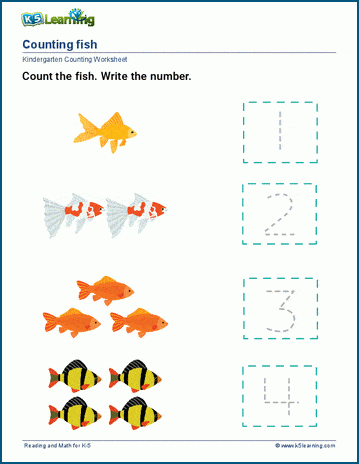## Color and count practice

Students read a number between one and five, and color the corresponding pictures in these worksheets. This is a simple number recognition and counting exercise.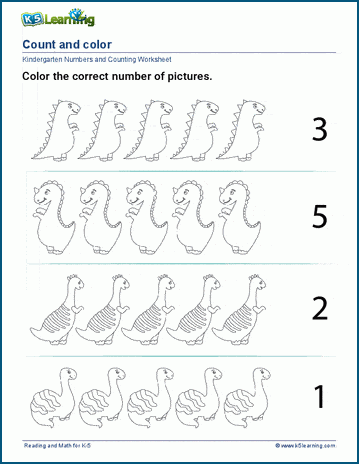## Counting like items

These sorting worksheets have students count the same items into groups. Worksheets for three groups and four groups are available for practice.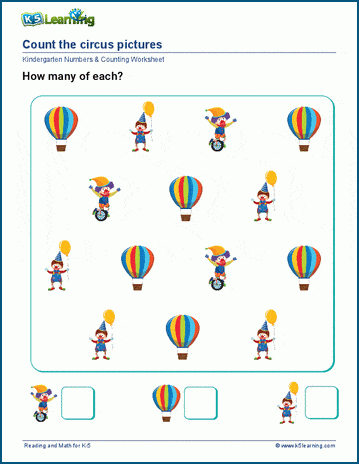## Practice counting and drawing to make 10

In these worksheets, students start by counting the number of objects. They then draw objects to make 10 on the page. Students will keep re-counting as they go.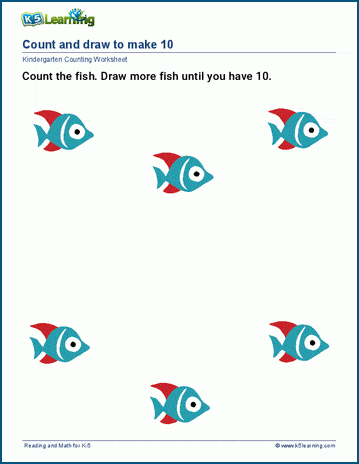## Crossing out practice

Students count each object as they cross them out and then write the number in the box in these worksheets.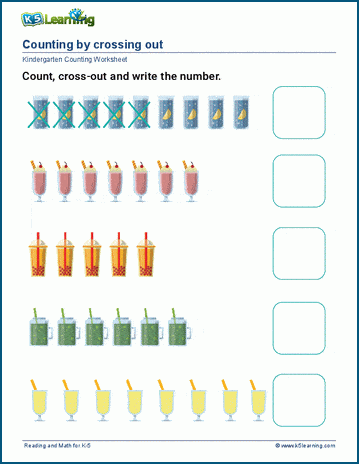## Count and match groups of items to numbers

Students first count the items in each box and then circle the corresponding number out of two choices. This is an exercise to help them count and recognize numbers.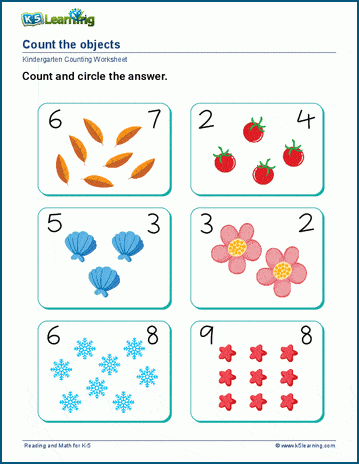## Practice counting up to 10 items

Similar to the crossing out worksheets, students count and write the number for each group of items. These worksheets are themed.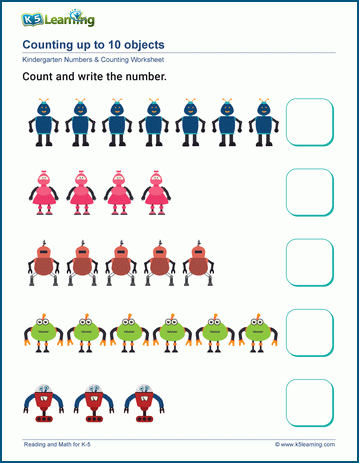## Counting and number lines worksheets

Students learn to use number lines in these worksheets.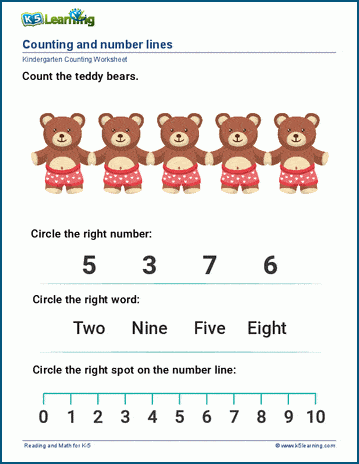## Count, then add or take away

Students are introduced to simple adding and subtracting by one.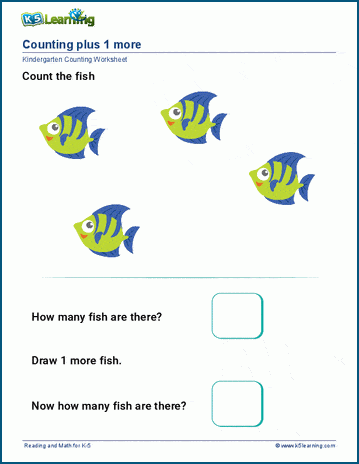## Practice counting objects in groups

Students count up to 10 of the same items and group them in these sorting worksheets.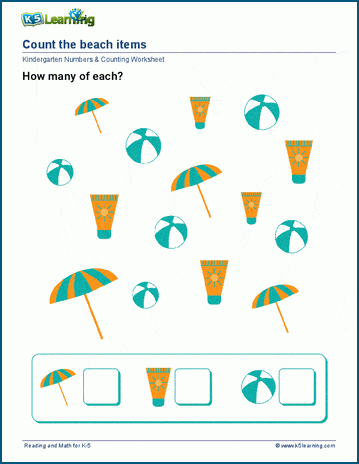Become a Member

This content is available to members only.